### Introduction

In this project a 2D frame to be modeled using SAP2000 software. It’s a four story with a 3-bay building which is to be built in an area of clay soil with soft rock within a seismic zone 3.

This building can be classified as a moment resisting frame. First and second floors have (30x35) cm beams and (50x50) cm columns, third and fourth floors have same previous beams dimensions and (40x50) cm columns.

### Numerical Model

In our numerical model equivalent lateral forces, spectral analysis and time history analysis have been used according to UBC-97 provisions.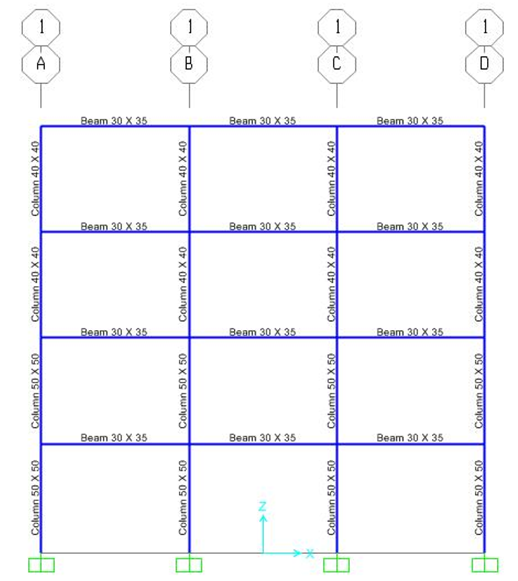Fig-1 : Numerical model of the frame

Gravity loads acting on the building are:

DL = 20 kN/m
LL = 15 kN/m

### Equivalent lateral force

In order to use this method of analysis using SAP2000 software these factors were inserted:

W = DL + 0.25LL

- Seismic importance factor (I)
I = 1 for special occupancy structures.

- Seismic zone factor (Z)
Z = 0.3 for zone 3.

- Structural system coefficient (R)
R = 8.5 for concrete moment resisting frame.

- Soil profile type (S)
SC for very dense soil (clay) and soft rock.

- Seismic coefficients:
Ct : 0.03 for concrete moment frames.

### Response spectrum analysis

Damping ratio (ξ) = 0.05

- Seismic coefficients:
Ca : 0.33 based on Z = 0.3 and soil profile SC .
Cv : 0.45 based on Z = 0.3 and soil profile SC .

period and acceleration we obtained from el-Centro earthquake data.

Fig-2 : Function graph of response spectrum

### Time history

Data was obtained from el-Centro earthquake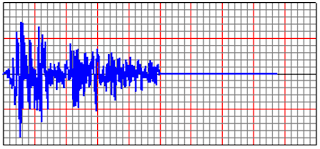Fig-3 : Function graph of time history

Analysis results and calculation

### Modal analysis

Modal analysis has been applied (for five modes) on the frame. Deformed shape, Natural frequency and period for each mode were as follows:

I. Mode 1 (T = 1.663 | f = 0.601)Fig-4 : Deformed shape of mode 1

II. Mode 2 (T = 0.472 | f = 2.120)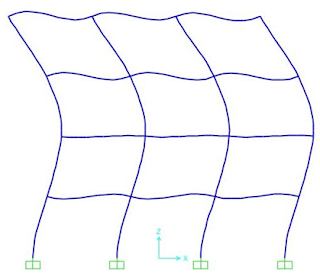Fig-5 : Deformed shape of mode 2

III. Mode 3 (T = 0.220 | f = 4.549)Fig-6 : Deformed shape of mode 3

IV. Mode 4 (T = 0.126 | f = 7.948)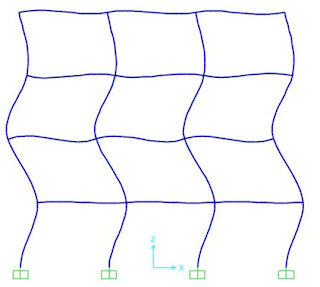Fig-7 : Deformed shape of mode 4

V. Mode 5 (T = 0.061 | f = 16.278)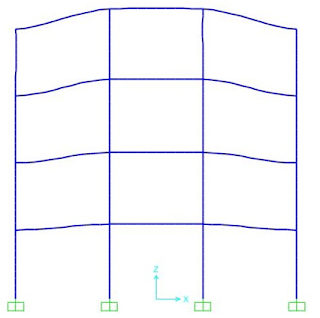Fig-8 : Deformed shape of mode 5

### Equivalent lateral force (Static analysis)Fig-9 : Shear envelope of equivalent lateral forces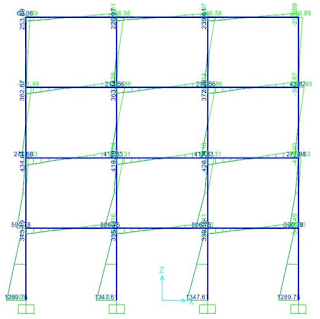Fig-10 : Moment envelope of equivalent lateral forces

### Time history analysisFig-11 : Shear envelope of Time history

Fig-12 : Moment envelope of Time history

Node 1:

Fig-13

Fig-14 : Time history of node 1